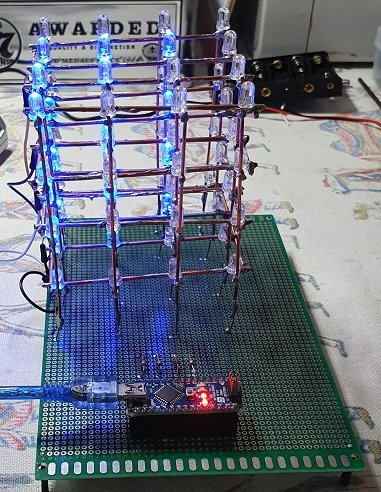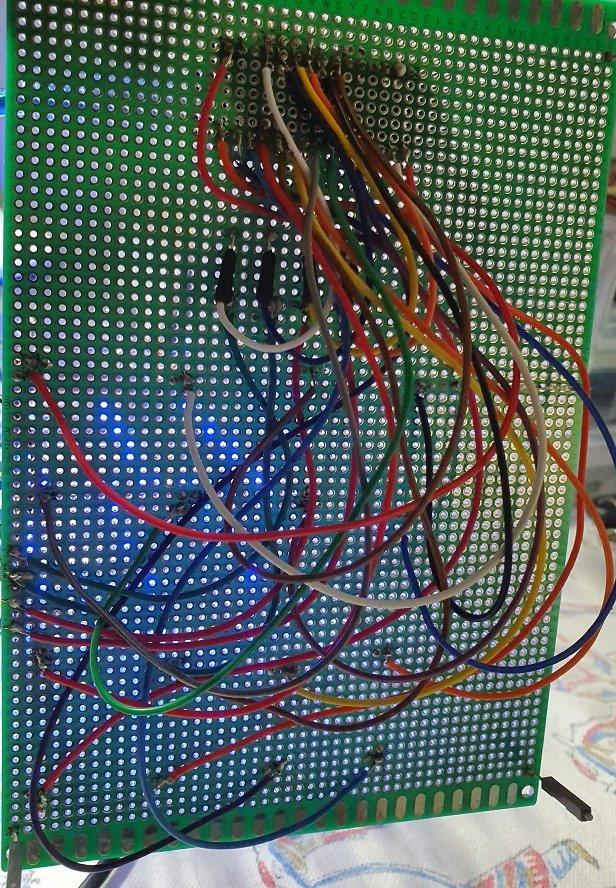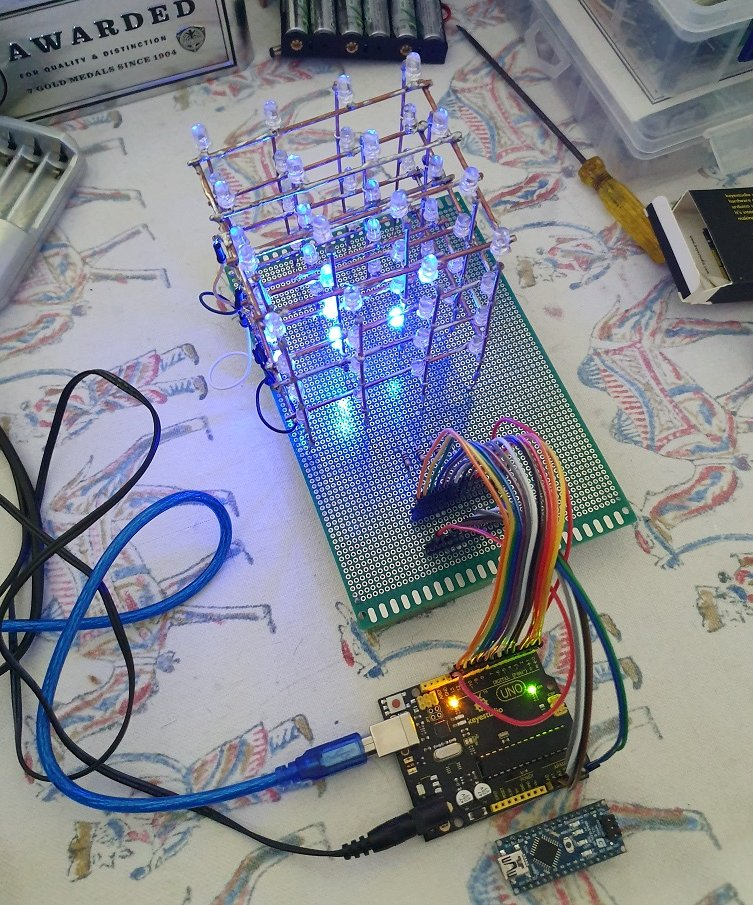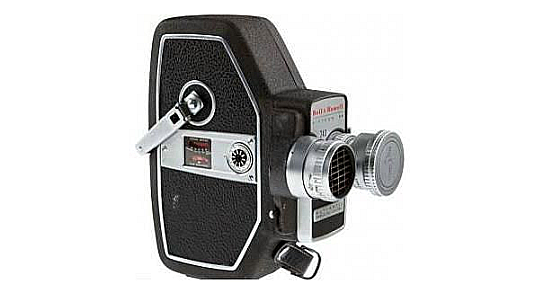Share My Creation 4x4x4 LED Cube

Discussion in 'B4R Share Your Creations' started by Johan Schoeman, Aug 18, 2019.

1. This is my attempt at building a 4x4x4 LED cube using an Arduino Nano (V3.0). It takes a lot of patience to build it with endless/non-stop soldering and testing. But what a pleasure to see it working once done!

Have used high brightness clear LED's emitting a blue light. All the wiring is at the bottom of the board other than the wires that connect to each of the 4 layers (LED anodes all connected to one another on each layer and all cathodes in each of the 16 columns connected to together.)

Pure magic when it is in a dark room and runs through the various subs that changes the display.Bottom of the board:Last edited: Aug 18, 2019
2. Sounds like it deserves a movie...

3. This is what I am just testing as code to switch 4x4x4 LED patterns in various ways in a 4x4x4 led cube.:
1. A range of random patterns.
2. Lighting of the cubes outline borders only
3. Using some inline C Code to display the outline of the cube
4. All anodes connected in plane/horizontal mode via 100 ohm resistors (x4) to A0,A1, A2, A3, A4
5. All cathode pins connected in columns and connected to pins A4, A5, D0, D1, D2, D3, D4, D5, D6, D7, D8 D9, D10, D12, D12, D13

Code:
#Region Project Attributes

#AutoFlushLogs: True

#CheckArrayBounds: True

#StackBufferSize: 300
#End Region

Sub Process_Globals

'These global variables will be declared once when the application starts.
'Public variables can be accessed from all modules.
Public Serial1 As Serial

Private layer(4As Pin 'Rows
Private column(16As Pin 'Columns

End Sub

Private Sub AppStart

'    Serial1.Initialize(115200)
'    Log("AppStart")

layer(
0).Initialize(column(0).A0, column(0).MODE_OUTPUT)
layer(
1).Initialize(column(1).A1, column(1).MODE_OUTPUT)
layer(
2).Initialize(column(2).A2, column(2).MODE_OUTPUT)
layer(
3).Initialize(column(3).A3, column(3).MODE_OUTPUT)

column(
0).Initialize(column(0).A4, column(0).MODE_OUTPUT)
column(
1).Initialize(column(1).A5, column(1).MODE_OUTPUT)

For i = 2 To 15
column(i).Initialize(i-
2, column(i).MODE_OUTPUT)

Next

turnEverythingOff
Delay(
1000)
RunNative(
"setup1"Null)

"myLooper")

End Sub

Sub myLooper

Dim p As Pin
p.Initialize(p.A6,p.MODE_INPUT)

Dim i As Int = Rnd(0,30)

If i < 6 Then i = 29

If i = 0 Then
anotherPropeller
turnEverythingOff
Delay(
50)

else if i = 1 Then
diagonalRectangle
turnEverythingOff
Delay(
50)

else if i = 2 Then
displayHorizontalFaceBottomToTop
turnEverythingOff
Delay(
50)

else if i = 3 Then
displayHorizontalFaceTopToBottom
turnEverythingOff
Delay(
50)

else if i = 4 Then
displayVerticalFaceBackToFront
turnEverythingOff
Delay(
50)

else if i = 5 Then
displayVerticalFaceFrontToBack
turnEverythingOff
Delay(
50)

else if i = 6 Then
displayVerticalFaceLeftToRight
turnEverythingOff
Delay(
50)

else if i = 7 Then
displayVerticalFaceRightToLeft
turnEverythingOff
Delay(
50)

else if i = 8 Then
flickerOff
turnEverythingOff
Delay(
50)

else if i = 9 Then
flickerOn
turnEverythingOff
Delay(
50)

else if i = 10 Then
goThroughAllLedsOneAtATime
turnEverythingOff
Delay(
50)

else if i = 11 Then
levo
turnEverythingOff
Delay(
50)

else if i = 12 Then
make_cross
turnEverythingOff
Delay(
50)

else if i = 13 Then
propeller
turnEverythingOff
Delay(
50)

else if i = 14 Then
randomColumn
turnEverythingOff
Delay(
50)

else if i = 15 Then
randomLed
turnEverythingOff
Delay(
50)

else if i = 16 Then
sequenceColumnRain
turnEverythingOff
Delay(
50)

else if i = 17 Then
snake
turnEverythingOff
Delay(
50)

else if i = 18 Then
spiralInAndOut
turnEverythingOff
Delay(
50)

else if i = 19 Then
switchAllVeryFast
turnEverythingOff
Delay(
50)

else if i = 20 Then
switchColumns
turnEverythingOff
Delay(
50)

else if i = 21 Then
switchRowsFrontToBackTopToBottom
turnEverythingOff
Delay(
50)

else if i = 22 Then
switchRowsRightToLeftTopToBottom
turnEverythingOff
Delay(
50)

else if i = 23 Then
switchSingleBackward

else if i = 24 Then
switchSingleForward

else if i = 25 Then
turnOnAndOffAllByLayerUpAndDownNotTimed
turnEverythingOff
Delay(
50)

else if i = 26 Then
drawOutline
turnEverythingOff
Delay(
50)

else if i = 27 Then
randomColumnRain
turnEverythingOff
Delay(
50)

else if i = 28 Then
sequenceColumnRain
turnEverythingOff
Delay(
50)

else if i = 29 Then
RunNative(
"loop1"Null)
turnEverythingOff
Delay(
50)

End If

End Sub

Public Sub turnEverythingOff

For n = 0 To 3
layer(n).DigitalWrite(
False)

Next

For n = 0 To 15
column(n).DigitalWrite(
True)

Next

End Sub

Sub turnEverythingOn

For i = 0 To 15
column(i).DigitalWrite(
False)

For j = 0 To 3
layer(j).DigitalWrite(
True)

Next

Next

End Sub

Sub switchSingleBackward

Dim x As Int = 5

For k = 0 To 20

For j = 0 To 3
layer(j).DigitalWrite(
True)

For i = 0 To 15
column(i).DigitalWrite(
False)
Delay(x)
column(i).DigitalWrite(
True)

Next
layer(j).DigitalWrite(
False)

Next

Next

End Sub

Sub switchSingleForward

Dim x As Int = 5

For k = 0 To 20

For j = 3 To 0 Step - 1
layer(j).DigitalWrite(
True)

For i = 15 To 0 Step - 1
column(i).DigitalWrite(
False)
Delay(x)
column(i).DigitalWrite(
True)

Next
layer(j).DigitalWrite(
False)

Next

Next

End Sub

Sub turnOnAndOffAllByLayerUpAndDownNotTimed

'turn everything on and off by layer up and down not TIMED

Dim x As Int = 25

For i = 10 To 1 Step - 1

turnEverythingOn

For j = 4 To 1

layer(j-
1).DigitalWrite(False)
Delay(x)

Next

For j = 0 To 3
layer(j).DigitalWrite(
True)
Delay(x)

Next

For j = 0 To 3
layer(j).DigitalWrite(
False)
Delay(x)

Next

For j = 4 To 1 Step - 1
layer(j-
1).DigitalWrite(True)
Delay(x)

Next

Next

End Sub

Sub flickerOn

Dim i As Int = 50

Do While i <> 0
turnEverythingOn
Delay(i)
turnEverythingOff
Delay(i)
i = i -
2

Loop
End Sub

Sub turnColumnsOff

For i = 0 To 15
column(i).DigitalWrite(
True)

Next

End Sub

Sub flickerOff

turnEverythingOn

For i = 2 To 20

If i <> 20 Then
turnEverythingOff
Delay(i+
25)
turnEverythingOn
Delay(i)

End If

Next
End Sub

Sub propeller

turnEverythingOff

Dim x As Int = 25

For y = 4 To 1 Step - 1

For i = 0 To 10
layer(y-
1).DigitalWrite(True)

'//a1
turnColumnsOff
column(
0).DigitalWrite(False)
column(
5).DigitalWrite(False)
column(
10).DigitalWrite(False)
column(
15).DigitalWrite(False)

Delay(x)

'//b1
turnColumnsOff
column(
4).DigitalWrite(False)
column(
5).DigitalWrite(False)
column(
10).DigitalWrite(False)
column(
11).DigitalWrite(False)
Delay(x)

'//c1
turnColumnsOff
column(
6).DigitalWrite(False)
column(
7).DigitalWrite(False)
column(
8).DigitalWrite(False)
column(
9).DigitalWrite(False)
Delay(x)

'//d1
turnColumnsOff
column(
3).DigitalWrite(False)
column(
6).DigitalWrite(False)
column(
9).DigitalWrite(False)
column(
12).DigitalWrite(False)
Delay(x)

'//d2
turnColumnsOff
column(
2).DigitalWrite(False)
column(
6).DigitalWrite(False)
column(
9).DigitalWrite(False)
column(
13).DigitalWrite(False)
Delay(x)

'//d3
turnColumnsOff
column(
1).DigitalWrite(False)
column(
5).DigitalWrite(False)
column(
10).DigitalWrite(False)
column(
14).DigitalWrite(False)
Delay(x)

Next

Next

turnColumnsOff
column(
14).DigitalWrite(False)
column(
10).DigitalWrite(False)
column(
5).DigitalWrite(False)
column(
1).DigitalWrite(False)
Delay(x)

End Sub

Sub anotherPropeller

turnEverythingOff

Dim x As Int = 20

For y = 0 To 3

For i = 0 To 15
layer(y).DigitalWrite(
True)

turnColumnsOff
column(
14).DigitalWrite(False)
column(
10).DigitalWrite(False)
column(
5).DigitalWrite(False)
column(
1).DigitalWrite(False)
Delay(x)

turnColumnsOff
column(
13).DigitalWrite(False)
column(
9).DigitalWrite(False)
column(
6).DigitalWrite(False)
column(
2).DigitalWrite(False)
Delay(x)

turnColumnsOff
column(
12).DigitalWrite(False)
column(
9).DigitalWrite(False)
column(
6).DigitalWrite(False)
column(
3).DigitalWrite(False)
Delay(x)

turnColumnsOff
column(
9).DigitalWrite(False)
column(
8).DigitalWrite(False)
column(
7).DigitalWrite(False)
column(
6).DigitalWrite(False)
Delay(x)

turnColumnsOff
column(
11).DigitalWrite(False)
column(
10).DigitalWrite(False)
column(
5).DigitalWrite(False)
column(
4).DigitalWrite(False)
Delay(x)

turnColumnsOff
column(
15).DigitalWrite(False)
column(
10).DigitalWrite(False)
column(
5).DigitalWrite(False)
column(
0).DigitalWrite(False)
Delay(x)

Next

Next
turnColumnsOff
column(
14).DigitalWrite(False)
column(
10).DigitalWrite(False)
column(
5).DigitalWrite(False)
column(
1).DigitalWrite(False)
Delay(x)

End Sub

Sub goThroughAllLedsOneAtATime

Dim x As Int = 2
turnEverythingOff

For y = 0 To 4

'//0-3
For count = 4 To 1 Step -1

layer(count-
1).DigitalWrite(True)

For i = 0 To 3
column(i).DigitalWrite(
False)

Delay(x)
column(i).DigitalWrite(
True)
Delay(x)

Next
layer(count-
1).DigitalWrite(False)

Next

'//4-7
For count = 0 To 3

layer(count).DigitalWrite(
True)

For i = 4 To 7

column(i).DigitalWrite(
False)
Delay(x)
column(i).DigitalWrite(
True)
Delay(x)

Next
layer(count).DigitalWrite(
False)

Next

'//8-11
For count = 4 To 1 Step - 1

layer(count-
1).DigitalWrite(True)

For i = 8 To 11

column(i).DigitalWrite(
False)
Delay(x)
column(i).DigitalWrite(
True)
Delay(x)

Next
layer(count-
1).DigitalWrite(False)

Next

'//12-15
For count = 0 To 3

layer(count).DigitalWrite(
True)

For i = 12 To 15

column(i).DigitalWrite(
False)
Delay(x)
column(i).DigitalWrite(
True)
Delay(x)

Next
layer(count).DigitalWrite(
False)

Next

Next

End Sub

Sub spiralInAndOut

turnEverythingOn

Dim x As Int = 20

For i = 0 To 5

'//spiral in clockwise
column(0).DigitalWrite(True)
Delay(x)
column(
1).DigitalWrite(True)
Delay(x)
column(
2).DigitalWrite(True)
Delay(x)
column(
3).DigitalWrite(True)
Delay(x)
column(
7).DigitalWrite(True)
Delay(x)
column(
11).DigitalWrite(True)
Delay(x)
column(
15).DigitalWrite(True)
Delay(x)
column(
14).DigitalWrite(True)
Delay(x)
column(
13).DigitalWrite(True)
Delay(x)
column(
12).DigitalWrite(True)
Delay(x)
column(
8).DigitalWrite(True)
Delay(x)
column(
4).DigitalWrite(True)
Delay(x)
column(
5).DigitalWrite(True)
Delay(x)
column(
6).DigitalWrite(True)
Delay(x)
column(
10).DigitalWrite(True)
Delay(x)
column(
9).DigitalWrite(True)
Delay(x)

'//spiral out counter clockwise
column(9).DigitalWrite(False)
Delay(x)
column(
10).DigitalWrite(False)
Delay(x)
column(
6).DigitalWrite(False)
Delay(x)
column(
5).DigitalWrite(False)
Delay(x)
column(
4).DigitalWrite(False)
Delay(x)
column(
8).DigitalWrite(False)
Delay(x)
column(
12).DigitalWrite(False)
Delay(x)
column(
13).DigitalWrite(False)
Delay(x)
column(
14).DigitalWrite(False)
Delay(x)
column(
15).DigitalWrite(False)
Delay(x)
column(
11).DigitalWrite(False)
Delay(x)
column(
7).DigitalWrite(False)
Delay(x)
column(
3).DigitalWrite(False)
Delay(x)
column(
2).DigitalWrite(False)
Delay(x)
column(
1).DigitalWrite(False)
Delay(x)
column(
0).DigitalWrite(False)
Delay(x)

'//spiral in counter clock wise
column(0).DigitalWrite(True)
Delay(x)
column(
4).DigitalWrite(True)
Delay(x)
column(
8).DigitalWrite(True)
Delay(x)
column(
12).DigitalWrite(True)
Delay(x)
column(
13).DigitalWrite(True)
Delay(x)
column(
14).DigitalWrite(True)
Delay(x)
column(
15).DigitalWrite(True)
Delay(x)
column(
11).DigitalWrite(True)
Delay(x)
column(
7).DigitalWrite(True)
Delay(x)
column(
3).DigitalWrite(True)
Delay(x)
column(
2).DigitalWrite(True)
Delay(x)
column(
1).DigitalWrite(True)
Delay(x)
column(
5).DigitalWrite(True)
Delay(x)
column(
9).DigitalWrite(True)
Delay(x)
column(
10).DigitalWrite(True)
Delay(x)
column(
6).DigitalWrite(True)
Delay(x)

'//spiral out clock wise
column(6).DigitalWrite(False)
Delay(x)
column(
10).DigitalWrite(False)
Delay(x)
column(
9).DigitalWrite(False)
Delay(x)
column(
5).DigitalWrite(False)
Delay(x)
column(
1).DigitalWrite(False)
Delay(x)
column(
2).DigitalWrite(False)
Delay(x)
column(
3).DigitalWrite(False)
Delay(x)
column(
7).DigitalWrite(False)
Delay(x)
column(
11).DigitalWrite(False)
Delay(x)
column(
15).DigitalWrite(False)
Delay(x)
column(
14).DigitalWrite(False)
Delay(x)
column(
13).DigitalWrite(False)
Delay(x)
column(
12).DigitalWrite(False)
Delay(x)
column(
8).DigitalWrite(False)
Delay(x)
column(
4).DigitalWrite(False)
Delay(x)
column(
0).DigitalWrite(False)
Delay(x)

Next

End Sub

Sub diagonalRectangle

Dim x As Int = 75
turnEverythingOff

For count = 0 To 4

'//top left
For i = 0 To 7
column(i).DigitalWrite(
False)

Next
layer(
3).DigitalWrite(True)
layer(
2).DigitalWrite(True)
Delay(x)
turnEverythingOff

'//middle middle
For i = 4 To 11
column(i).DigitalWrite(
False)

Next
layer(
1).DigitalWrite(True)
layer(
2).DigitalWrite(True)
Delay(x)
turnEverythingOff

'//bottom right
For i = 8 To 15
column(i).DigitalWrite(
False)

Next
layer(
0).DigitalWrite(True)
layer(
1).DigitalWrite(True)
Delay(x)
turnEverythingOff

'//bottom middle
For i = 4 To 11
column(i).DigitalWrite(
False)

Next
layer(
0).DigitalWrite(True)
layer(
1).DigitalWrite(True)
Delay(x)
turnEverythingOff

'//bottom left
For i = 0 To 7
column(i).DigitalWrite(
False)

Next
layer(
0).DigitalWrite(True)
layer(
1).DigitalWrite(True)
Delay(x)
turnEverythingOff

'//middle middle
For i = 4 To 11
column(i).DigitalWrite(
False)

Next
layer(
1).DigitalWrite(True)
layer(
2).DigitalWrite(True)
Delay(x)
turnEverythingOff

'//top right
For i = 8 To 15
column(i).DigitalWrite(
False)

Next
layer(
2).DigitalWrite(True)
layer(
3).DigitalWrite(True)
Delay(x)
turnEverythingOff

'//top middle
For i = 4 To 11
column(i).DigitalWrite(
False)

Next
layer(
2).DigitalWrite(True)
layer(
3).DigitalWrite(True)
Delay(x)
turnEverythingOff

Next

'//top left
For i = 0 To 7
column(i).DigitalWrite(
False)

Next
layer(
3).DigitalWrite(True)
layer(
2).DigitalWrite(True)
Delay(x)
turnEverythingOff

End Sub

Sub displayVerticalFaceFrontToBack

For cnt = 0 To 5

For i = 0 To 3
layer(i).DigitalWrite(
True)

Next

For j = 0 To 3
column(j*
4).DigitalWrite(False)
column(j*
4+1).DigitalWrite(False)
column(j*
4+2).DigitalWrite(False)
column(j*
4+3).DigitalWrite(False)
Delay(
150)
column(j*
4).DigitalWrite(True)
column(j*
4+1).DigitalWrite(True)
column(j*
4+2).DigitalWrite(True)
column(j*
4+3).DigitalWrite(True)

Next

turnEverythingOff

Next

End Sub

Sub displayVerticalFaceLeftToRight

For cnt = 0 To 5

For i = 0 To 3
layer(i).DigitalWrite(
True)

Next

For j = 3 To 0 Step - 1
column(j).DigitalWrite(
False)
column(j+
4).DigitalWrite(False)
column(j+
8).DigitalWrite(False)
column(j+
12).DigitalWrite(False)
Delay(
250)
column(j).DigitalWrite(
True)
column(j+
4).DigitalWrite(True)
column(j+
8).DigitalWrite(True)
column(j+
12).DigitalWrite(True)

Next

turnEverythingOff

Next

End Sub

Sub displayHorizontalFaceTopToBottom

For k = 0 To 5

For i = 3 To 0 Step - 1
layer(i).DigitalWrite(
True)

For j = 0 To 15
column(j).DigitalWrite(
False)

Next
Delay(
100)
layer(i).DigitalWrite(
False)

For j = 0 To 15
column(j).DigitalWrite(
True)

Next

Next

Next

End Sub

Sub displayVerticalFaceBackToFront

For cnt = 0 To 5

For i = 0 To 3
layer(i).DigitalWrite(
True)

Next

For j = 3 To 0 Step - 1
column(j*
4).DigitalWrite(False)
column(j*
4+1).DigitalWrite(False)
column(j*
4+2).DigitalWrite(False)
column(j*
4+3).DigitalWrite(False)
Delay(
200)
column(j*
4).DigitalWrite(True)
column(j*
4+1).DigitalWrite(True)
column(j*
4+2).DigitalWrite(True)
column(j*
4+3).DigitalWrite(True)

Next
turnEverythingOff

Next

End Sub

Sub displayVerticalFaceRightToLeft

For cnt = 0 To 5

For i = 0 To 3
layer(i).DigitalWrite(
True)

Next

For j = 0 To 3
column(j).DigitalWrite(
False)
column(j+
4).DigitalWrite(False)
column(j+
8).DigitalWrite(False)
column(j+
12).DigitalWrite(False)
Delay(
200)
column(j).DigitalWrite(
True)
column(j+
4).DigitalWrite(True)
column(j+
8).DigitalWrite(True)
column(j+
12).DigitalWrite(True)

Next
turnEverythingOff

Next

End Sub

Sub displayHorizontalFaceBottomToTop

For k = 0 To 5

For i = 0 To 3
layer(i).DigitalWrite(
True)

For j = 0 To 15
column(j).DigitalWrite(
False)

Next
Delay(
100)
layer(i).DigitalWrite(
False)

For j = 0 To 15
column(j).DigitalWrite(
True)

Next

Next

Next

End Sub

Sub switchColumns

For k = 0 To 5

For i = 0 To 3
layer(i).DigitalWrite(
True)

Next

For j = 0 To 15
column(j).DigitalWrite(
False)
Delay(
50)
column(j).DigitalWrite(
True)

Next

For i = 0 To 3
layer(i).DigitalWrite(
False)

Next

Next

End Sub

Sub switchRowsFrontToBackTopToBottom

For k = 0 To 5

For i = 3 To 0 Step - 1
layer(i).DigitalWrite(
True)

For j = 0 To 12 Step 4
column(j).DigitalWrite(
False)
column(j+
1).DigitalWrite(False)
column(j+
2).DigitalWrite(False)
column(j+
3).DigitalWrite(False)
Delay(
50)
column(j).DigitalWrite(
True)
column(j+
1).DigitalWrite(True)
column(j+
2).DigitalWrite(True)
column(j+
3).DigitalWrite(True)

Next

layer(i).DigitalWrite(
False)

Next

Next

End Sub

Sub switchRowsRightToLeftTopToBottom

For k = 0 To 5

For i = 3 To 0 Step - 1
layer(i).DigitalWrite(
True)

For j = 0 To 3
column(j).DigitalWrite(
False)
column(j+
4).DigitalWrite(False)
column(j+
8).DigitalWrite(False)
column(j+
12).DigitalWrite(False)
Delay(
75)
column(j).DigitalWrite(
True)
column(j+
4).DigitalWrite(True)
column(j+
8).DigitalWrite(True)
column(j+
12).DigitalWrite(True)

Next

layer(i).DigitalWrite(
False)

Next

Next

End Sub

Sub levo

For m = 0 To 4

Dim k As Int = 4

Dim p As Int = 0

For i = 3 To 0 Step - 1
layer(i).DigitalWrite(
True)

Next

Do While p<4

For j = k-4 To k-1
column(j).DigitalWrite(
False)

Next

Delay(
100)

For j = k-4 To k-1
column(j).DigitalWrite(
False)

Next
k = k +
4
p = p +
1

Loop

turnEverythingOff
Delay(
50)

Next

End Sub

Sub snake

For k = 0 To 4

'layer 3

layer(
3).DigitalWrite(True)

For i = 0 To 3
column(i).DigitalWrite(
False)
Delay(
20)
column(i).DigitalWrite(
True)

Next

For i = 7 To 4 Step - 1
column(i).DigitalWrite(
False)
Delay(
20)
column(i).DigitalWrite(
True)

Next

For i = 8 To 11
column(i).DigitalWrite(
False)
Delay(
20)
column(i).DigitalWrite(
True)

Next

For i = 15 To 12 Step - 1
column(i).DigitalWrite(
False)
Delay(
20)
column(i).DigitalWrite(
True)

Next

layer(
3).DigitalWrite(False)

'layer 2

layer(
2).DigitalWrite(True)

For i = 12 To 15
column(i).DigitalWrite(
False)
Delay(
20)
column(i).DigitalWrite(
True)

Next

For i = 11 To 8 Step - 1
column(i).DigitalWrite(
False)
Delay(
20)
column(i).DigitalWrite(
True)

Next

For i = 4 To 7
column(i).DigitalWrite(
False)
Delay(
20)
column(i).DigitalWrite(
True)

Next

For i = 3 To 0 Step - 1
column(i).DigitalWrite(
False)
Delay(
20)
column(i).DigitalWrite(
True)

Next

layer(
2).DigitalWrite(False)

'layer 1

layer(
1).DigitalWrite(True)

For i = 0 To 3
column(i).DigitalWrite(
False)
Delay(
20)
column(i).DigitalWrite(
True)

Next

For i = 7 To 4 Step - 1
column(i).DigitalWrite(
False)
Delay(
20)
column(i).DigitalWrite(
True)

Next

For i = 8 To 11
column(i).DigitalWrite(
False)
Delay(
20)
column(i).DigitalWrite(
True)

Next

For i = 15 To 12 Step - 1
column(i).DigitalWrite(
False)
Delay(
20)
column(i).DigitalWrite(
True)

Next

layer(
1).DigitalWrite(False)

'layer 0

layer(
0).DigitalWrite(True)

For i = 12 To 15
column(i).DigitalWrite(
False)
Delay(
20)
column(i).DigitalWrite(
True)

Next

For i = 11 To 8 Step - 1
column(i).DigitalWrite(
False)
Delay(
20)
column(i).DigitalWrite(
True)

Next

For i = 4 To 7
column(i).DigitalWrite(
False)
Delay(
20)
column(i).DigitalWrite(
True)

Next

For i = 3 To 0 Step - 1
column(i).DigitalWrite(
False)
Delay(
20)
column(i).DigitalWrite(
True)

Next

layer(
0).DigitalWrite(False)

Next

End Sub

Sub make_cross

Dim x As Int = 202

For k = 0 To 200

For i = 0 To 3
layer(i).DigitalWrite(
True)

Next

column(
3).DigitalWrite(False)
column(
6).DigitalWrite(False)
column(
9).DigitalWrite(False)
column(
12).DigitalWrite(False)

Delay(x)

column(
3).DigitalWrite(True)
column(
6).DigitalWrite(True)
column(
9).DigitalWrite(True)
column(
12).DigitalWrite(True)

column(
0).DigitalWrite(False)
column(
5).DigitalWrite(False)
column(
10).DigitalWrite(False)
column(
15).DigitalWrite(False)

Delay(x)

column(
0).DigitalWrite(True)
column(
5).DigitalWrite(True)
column(
10).DigitalWrite(True)
column(
15).DigitalWrite(True)

x = x -
1

Next

End Sub

Sub randomLed

For k = 0 To 1000

Dim p As Pin
p.Initialize(p.A6,p.MODE_INPUT)

Dim i As Byte = Rnd(04)

Dim j As Byte = Rnd(0,16)

layer(i).DigitalWrite(
True)
column(j).DigitalWrite(
False)
Delay(
10)
turnEverythingOff

Next

End Sub

Sub randomColumn

Dim x As Byte = 1002

Dim prevval, newval As Byte = 0

Dim p As Pin
p.Initialize(p.A6,p.MODE_INPUT)

For i = 0 To 3
layer(i).DigitalWrite(
True)

Next

For k = 0 To 100

Dim newval As Byte = Rnd(0,16)

If newval <> prevval Then

column(newval).DigitalWrite(
False)
Delay(x)
x = x - k
column(newval).DigitalWrite(
True)
prevval = newval

End If

Next

End Sub

Sub sequenceColumnRain

For k = 0 To 4

For i = 0 To 15
column(i).DigitalWrite(
False)

For j = 3 To 0 Step - 1
layer(j).DigitalWrite(
True)
Delay(
50)
layer(j).DigitalWrite(
False)

Next
column(i).DigitalWrite(
True)

Next
turnEverythingOff

Next

End Sub

Sub switchAllVeryFast

Dim x As Int = 400

For k = 0 To 100

For i = 0 To 3
layer(i).DigitalWrite(
True)

For j = 0 To 15
column(j).DigitalWrite(
False)
DelayMicroseconds(x)
x = x +
10
column(j).DigitalWrite(
True)

Next
layer(i).DigitalWrite(
False)

Next

Next

End Sub

Sub drawOutline

For m = 0 To 3

Dim k As Int  = 2

Do While k < 50

For i = 3 To 0 Step - 1
layer(i).DigitalWrite(
True)

For j = 0 To 15
column(j).DigitalWrite(
False)

Next

Next
Delay(k+
2)

For i = 3 To 0 Step - 1
layer(i).DigitalWrite(
False)

For j = 0 To 15
column(j).DigitalWrite(
True)

Next

Next
Delay(k)

k = k +
1

Loop

Next

End Sub

Sub randomColumnRain

Dim p As Pin
p.Initialize(p.A6,p.MODE_INPUT)

For m = 0 To 100

Dim r As Byte = Rnd(0,4)

Dim k As Byte = Rnd(0,16)

layer(r).DigitalWrite(
True)
column(k).DigitalWrite(
False)
Delay(
100)

layer(r).DigitalWrite(
False)
column(k).DigitalWrite(
True)
Delay(
50)

Next

End Sub

#if C

//4x4x4 LED Cube Show 1
//This one was written by someone else, i dont know who, however if you want credit, message me!

#include <avr/pgmspace.h> // allows use of PROGMEM to store patterns in flash

#define CUBESIZE 4
#define PLANESIZE CUBESIZE*CUBESIZE
#define PLANETIME 2000 // time each plane is displayed in us -> 100 Hz refresh
#define TIMECONST 10// multiplies DisplayTime to get ms - why not =100?

// LED Pattern Table in PROGMEM - last column is display time in 100ms units
// TODO this could be a lot more compact but not with binary pattern representation
const unsigned char PROGMEM PatternTable[] = {
// blink on and off
//Floor:
//1            1         1          1           2          2          2           2           3         3           3          3          4           4          4          4
//Row:
//1            2         3          4           1          2          3           4           1         2           3          4           1          2          3          4

/****************************REPLACE THIS WITH THE GENERATED CODE****************************/

//0 = on, 1 = off
//this line lights up the edges of the cube
B0000,B0110,B0110,B0000   ,B0110,B1111,B1111,B0110   ,B0110,B1111,B1111,B0110   ,B0000,B0110,B0110,B0000   , 200,
B1111,B1001,B1001,B1111   ,B1001,B0000,B0000,B1001   ,B1001,B0000,B0000,B1001   ,B1111,B1001,B1001,B1111   , 200,
B0000,B0110,B0110,B0000   ,B0110,B1111,B1111,B0110   ,B0110,B1111,B1111,B0110   ,B0000,B0110,B0110,B0000   , 150,
B1111,B1001,B1001,B1111   ,B1001,B0000,B0000,B1001   ,B1001,B0000,B0000,B1001   ,B1111,B1001,B1001,B1111   , 150,
B0000,B0110,B0110,B0000   ,B0110,B1111,B1111,B0110   ,B0110,B1111,B1111,B0110   ,B0000,B0110,B0110,B0000   , 100,
B1111,B1001,B1001,B1111   ,B1001,B0000,B0000,B1001   ,B1001,B0000,B0000,B1001   ,B1111,B1001,B1001,B1111   , 100,
B0000,B0110,B0110,B0000   ,B0110,B1111,B1111,B0110   ,B0110,B1111,B1111,B0110   ,B0000,B0110,B0110,B0000   , 75,
B1111,B1001,B1001,B1111   ,B1001,B0000,B0000,B1001   ,B1001,B0000,B0000,B1001   ,B1111,B1001,B1001,B1111   , 75,
B0000,B0110,B0110,B0000   ,B0110,B1111,B1111,B0110   ,B0110,B1111,B1111,B0110   ,B0000,B0110,B0110,B0000   , 50,
B1111,B1001,B1001,B1111   ,B1001,B0000,B0000,B1001   ,B1001,B0000,B0000,B1001   ,B1111,B1001,B1001,B1111   , 50,
B0000,B0110,B0110,B0000   ,B0110,B1111,B1111,B0110   ,B0110,B1111,B1111,B0110   ,B0000,B0110,B0110,B0000   , 25,
B1111,B1001,B1001,B1111   ,B1001,B0000,B0000,B1001   ,B1001,B0000,B0000,B1001   ,B1111,B1001,B1001,B1111   , 25,
B0000,B0110,B0110,B0000   ,B0110,B1111,B1111,B0110   ,B0110,B1111,B1111,B0110   ,B0000,B0110,B0110,B0000   , 10,
B1111,B1001,B1001,B1111   ,B1001,B0000,B0000,B1001   ,B1001,B0000,B0000,B1001   ,B1111,B1001,B1001,B1111   , 10,
B0000,B0110,B0110,B0000   ,B0110,B1111,B1111,B0110   ,B0110,B1111,B1111,B0110   ,B0000,B0110,B0110,B0000   , 5,
B1111,B1001,B1001,B1111   ,B1001,B0000,B0000,B1001   ,B1001,B0000,B0000,B1001   ,B1111,B1001,B1001,B1111   , 5,
B0000,B0110,B0110,B0000   ,B0110,B1111,B1111,B0110   ,B0110,B1111,B1111,B0110   ,B0000,B0110,B0110,B0000   , 2,
B1111,B1001,B1001,B1111   ,B1001,B0000,B0000,B1001   ,B1001,B0000,B0000,B1001   ,B1111,B1001,B1001,B1111   , 2,
B0000,B0110,B0110,B0000   ,B0110,B1111,B1111,B0110   ,B0110,B1111,B1111,B0110   ,B0000,B0110,B0110,B0000   , 2,
B1111,B1001,B1001,B1111   ,B1001,B0000,B0000,B1001   ,B1001,B0000,B0000,B1001   ,B1111,B1001,B1001,B1111   , 2,
B0000,B0110,B0110,B0000   ,B0110,B1111,B1111,B0110   ,B0110,B1111,B1111,B0110   ,B0000,B0110,B0110,B0000   , 2,
B1111,B1001,B1001,B1111   ,B1001,B0000,B0000,B1001   ,B1001,B0000,B0000,B1001   ,B1111,B1001,B1001,B1111   , 2,
B0000,B0110,B0110,B0000   ,B0110,B1111,B1111,B0110   ,B0110,B1111,B1111,B0110   ,B0000,B0110,B0110,B0000   , 2,
B1111,B1001,B1001,B1111   ,B1001,B0000,B0000,B1001   ,B1001,B0000,B0000,B1001   ,B1111,B1001,B1001,B1111   , 2,
B0000,B0110,B0110,B0000   ,B0110,B1111,B1111,B0110   ,B0110,B1111,B1111,B0110   ,B0000,B0110,B0110,B0000   , 2,
B1111,B1001,B1001,B1111   ,B1001,B0000,B0000,B1001   ,B1001,B0000,B0000,B1001   ,B1111,B1001,B1001,B1111   , 2,
B0000,B0110,B0110,B0000   ,B0110,B1111,B1111,B0110   ,B0110,B1111,B1111,B0110   ,B0000,B0110,B0110,B0000   , 2,
B1111,B1001,B1001,B1111   ,B1001,B0000,B0000,B1001   ,B1001,B0000,B0000,B1001   ,B1111,B1001,B1001,B1111   , 2,
B0000,B0110,B0110,B0000   ,B0110,B1111,B1111,B0110   ,B0110,B1111,B1111,B0110   ,B0000,B0110,B0110,B0000   , 2,
B1111,B1001,B1001,B1111   ,B1001,B0000,B0000,B1001   ,B1001,B0000,B0000,B1001   ,B1111,B1001,B1001,B1111   , 2,
B0000,B0110,B0110,B0000   ,B0110,B1111,B1111,B0110   ,B0110,B1111,B1111,B0110   ,B0000,B0110,B0110,B0000   , 2,
B1111,B1001,B1001,B1111   ,B1001,B0000,B0000,B1001   ,B1001,B0000,B0000,B1001   ,B1111,B1001,B1001,B1111   , 2,
B0000,B0110,B0110,B0000   ,B0110,B1111,B1111,B0110   ,B0110,B1111,B1111,B0110   ,B0000,B0110,B0110,B0000   , 2,
B1111,B1001,B1001,B1111   ,B1001,B0000,B0000,B1001   ,B1001,B0000,B0000,B1001   ,B1111,B1001,B1001,B1111   , 2,
B0000,B0110,B0110,B0000   ,B0110,B1111,B1111,B0110   ,B0110,B1111,B1111,B0110   ,B0000,B0110,B0110,B0000   , 2,
B1111,B1001,B1001,B1111   ,B1001,B0000,B0000,B1001   ,B1001,B0000,B0000,B1001   ,B1111,B1001,B1001,B1111   , 2,
B0000,B0110,B0110,B0000   ,B0110,B1111,B1111,B0110   ,B0110,B1111,B1111,B0110   ,B0000,B0110,B0110,B0000   , 2,
B1111,B1001,B1001,B1111   ,B1001,B0000,B0000,B1001   ,B1001,B0000,B0000,B1001   ,B1111,B1001,B1001,B1111   , 2,
B0000,B0110,B0110,B0000   ,B0110,B1111,B1111,B0110   ,B0110,B1111,B1111,B0110   ,B0000,B0110,B0110,B0000   , 2,
B1111,B1001,B1001,B1111   ,B1001,B0000,B0000,B1001   ,B1001,B0000,B0000,B1001   ,B1111,B1001,B1001,B1111   , 2,
B0000,B0110,B0110,B0000   ,B0110,B1111,B1111,B0110   ,B0110,B1111,B1111,B0110   ,B0000,B0110,B0110,B0000   , 2,
B1111,B1001,B1001,B1111   ,B1001,B0000,B0000,B1001   ,B1001,B0000,B0000,B1001   ,B1111,B1001,B1001,B1111   , 2,
B0000,B0110,B0110,B0000   ,B0110,B1111,B1111,B0110   ,B0110,B1111,B1111,B0110   ,B0000,B0110,B0110,B0000   , 2,
B1111,B1001,B1001,B1111   ,B1001,B0000,B0000,B1001   ,B1001,B0000,B0000,B1001   ,B1111,B1001,B1001,B1111   , 2,
B0000,B0110,B0110,B0000   ,B0110,B1111,B1111,B0110   ,B0110,B1111,B1111,B0110   ,B0000,B0110,B0110,B0000   , 2,
B1111,B1001,B1001,B1111   ,B1001,B0000,B0000,B1001   ,B1001,B0000,B0000,B1001   ,B1111,B1001,B1001,B1111   , 2,
B0000,B0110,B0110,B0000   ,B0110,B1111,B1111,B0110   ,B0110,B1111,B1111,B0110   ,B0000,B0110,B0110,B0000   , 2,
B1111,B1001,B1001,B1111   ,B1001,B0000,B0000,B1001   ,B1001,B0000,B0000,B1001   ,B1111,B1001,B1001,B1111   , 2,
B0000,B0110,B0110,B0000   ,B0110,B1111,B1111,B0110   ,B0110,B1111,B1111,B0110   ,B0000,B0110,B0110,B0000   , 2,
B1111,B1001,B1001,B1111   ,B1001,B0000,B0000,B1001   ,B1001,B0000,B0000,B1001   ,B1111,B1001,B1001,B1111   , 2,
B0000,B0110,B0110,B0000   ,B0110,B1111,B1111,B0110   ,B0110,B1111,B1111,B0110   ,B0000,B0110,B0110,B0000   , 2,
B1111,B1001,B1001,B1111   ,B1001,B0000,B0000,B1001   ,B1001,B0000,B0000,B1001   ,B1111,B1001,B1001,B1111   , 2,
B0000,B0110,B0110,B0000   ,B0110,B1111,B1111,B0110   ,B0110,B1111,B1111,B0110   ,B0000,B0110,B0110,B0000   , 2,
B1111,B1001,B1001,B1111   ,B1001,B0000,B0000,B1001   ,B1001,B0000,B0000,B1001   ,B1111,B1001,B1001,B1111   , 2,
B0000,B0110,B0110,B0000   ,B0110,B1111,B1111,B0110   ,B0110,B1111,B1111,B0110   ,B0000,B0110,B0110,B0000   , 2,
B1111,B1001,B1001,B1111   ,B1001,B0000,B0000,B1001   ,B1001,B0000,B0000,B1001   ,B1111,B1001,B1001,B1111   , 2,
B0000,B0110,B0110,B0000   ,B0110,B1111,B1111,B0110   ,B0110,B1111,B1111,B0110   ,B0000,B0110,B0110,B0000   , 2,
B1111,B1001,B1001,B1111   ,B1001,B0000,B0000,B1001   ,B1001,B0000,B0000,B1001   ,B1111,B1001,B1001,B1111   , 2,
B0000,B0110,B0110,B0000   ,B0110,B1111,B1111,B0110   ,B0110,B1111,B1111,B0110   ,B0000,B0110,B0110,B0000   , 2,
B1111,B1001,B1001,B1111   ,B1001,B0000,B0000,B1001   ,B1001,B0000,B0000,B1001   ,B1111,B1001,B1001,B1111   , 2,
B0000,B0110,B0110,B0000   ,B0110,B1111,B1111,B0110   ,B0110,B1111,B1111,B0110   ,B0000,B0110,B0110,B0000   , 2,
B1111,B1001,B1001,B1111   ,B1001,B0000,B0000,B1001   ,B1001,B0000,B0000,B1001   ,B1111,B1001,B1001,B1111   , 2,
B0000,B0110,B0110,B0000   ,B0110,B1111,B1111,B0110   ,B0110,B1111,B1111,B0110   ,B0000,B0110,B0110,B0000   , 2,
B1111,B1001,B1001,B1111   ,B1001,B0000,B0000,B1001   ,B1001,B0000,B0000,B1001   ,B1111,B1001,B1001,B1111   , 2,
B0000,B0110,B0110,B0000   ,B0110,B1111,B1111,B0110   ,B0110,B1111,B1111,B0110   ,B0000,B0110,B0110,B0000   , 2,
B1111,B1001,B1001,B1111   ,B1001,B0000,B0000,B1001   ,B1001,B0000,B0000,B1001   ,B1111,B1001,B1001,B1111   , 2,
B0000,B0110,B0110,B0000   ,B0110,B1111,B1111,B0110   ,B0110,B1111,B1111,B0110   ,B0000,B0110,B0110,B0000   , 2,
B1111,B1001,B1001,B1111   ,B1001,B0000,B0000,B1001   ,B1001,B0000,B0000,B1001   ,B1111,B1001,B1001,B1111   , 2,
B0000,B0110,B0110,B0000   ,B0110,B1111,B1111,B0110   ,B0110,B1111,B1111,B0110   ,B0000,B0110,B0110,B0000   , 2,
B1111,B1001,B1001,B1111   ,B1001,B0000,B0000,B1001   ,B1001,B0000,B0000,B1001   ,B1111,B1001,B1001,B1111   , 2,
B0000,B0110,B0110,B0000   ,B0110,B1111,B1111,B0110   ,B0110,B1111,B1111,B0110   ,B0000,B0110,B0110,B0000   , 2,
B1111,B1001,B1001,B1111   ,B1001,B0000,B0000,B1001   ,B1001,B0000,B0000,B1001   ,B1111,B1001,B1001,B1111   , 2,
B0000,B0110,B0110,B0000   ,B0110,B1111,B1111,B0110   ,B0110,B1111,B1111,B0110   ,B0000,B0110,B0110,B0000   , 2,
B1111,B1001,B1001,B1111   ,B1001,B0000,B0000,B1001   ,B1001,B0000,B0000,B1001   ,B1111,B1001,B1001,B1111   , 2,

//another pattern
B1111,B1111,B1111,B0000   ,B1111,B1111,B1111,B0000   ,B1111,B1111,B1111,B0000   ,B1111,B1111,B1111,B0000   , 35,
B1111,B1111,B0000,B1111   ,B1111,B1111,B0000,B1111   ,B1111,B1111,B0000,B1111   ,B1111,B1111,B0000,B1111   , 35,
B1111,B0000,B1111,B1111   ,B1111,B0000,B1111,B1111   ,B1111,B0000,B1111,B1111   ,B1111,B0000,B1111,B1111   , 35,
B0000,B1111,B1111,B1111   ,B0000,B1111,B1111,B1111   ,B0000,B1111,B1111,B1111   ,B0000,B1111,B1111,B1111   , 35,

//switch all off
B1111,B1111,B1111,B1111   ,B1111,B1111,B1111,B1111   ,B1111,B1111,B1111,B1111   ,B1111,B1111,B1111,B1111   , 50,

B0000,B1111,B1111,B1111   ,B0000,B1111,B1111,B1111   ,B0000,B1111,B1111,B1111   ,B0000,B1111,B1111,B1111   , 70,
B1111,B0000,B1111,B1111   ,B1111,B0000,B1111,B1111   ,B1111,B0000,B1111,B1111   ,B1111,B0000,B1111,B1111   , 70,
B1111,B1111,B0000,B1111   ,B1111,B1111,B0000,B1111   ,B1111,B1111,B0000,B1111   ,B1111,B1111,B0000,B1111   , 70,
B1111,B1111,B1111,B0000   ,B1111,B1111,B1111,B0000   ,B1111,B1111,B1111,B0000   ,B1111,B1111,B1111,B0000   , 70,

//
//switch all off
B1111,B1111,B1111,B1111   ,B1111,B1111,B1111,B1111   ,B1111,B1111,B1111,B1111   ,B1111,B1111,B1111,B1111   , 50,
B0100,B1010,B1001,B1011   ,B1111,B1101,B1111,B1101   ,B0010,B0110,B0001,B1111   ,B1101,B1001,B1111,B0110   , 35,
B1111,B1111,B1111,B1111   ,B1111,B1111,B1111,B1111   ,B1111,B1111,B1111,B1111   ,B1111,B1111,B1111,B1111   , 50,
B1011,B1001,B1100,B1010   ,B0110,B0110,B1011,B1010   ,B0101,B1010,B1101,B0101   ,B1010,B1101,B0011,B0111   , 35,

// this is a dummy element for end of table (duration=0) aka !!!DO NOT TOUCH!!!
B0000, B0000, B0000, B0000, B0000, B0000, B0000, B0000, B0000, B0000, B0000, B0000, B0000, B0000, B0000, B0000, 0};

/*
** Defining pins in array makes it easier to rearrange how cube is wired
** Adjust numbers here until LEDs flash in order - L to R, T to B
** Note that analog inputs 0-5 are also digital outputs 14-19!
** Pin DigitalOut0 (serial RX) and AnalogIn5 are left open for future apps
*/

//    int LEDPin[] = {A4, A5, 0, 1, 2, 3, 4, 5, 6, 7, 8, 9, 10, 11, 12, 13};
int LEDPin[] = {13, 12, 11, 10, 9, 8, 7, 6, 5, 4, 3, 2, 1, 0, A5, A4};
int PlanePin[] = {A0, A1, A2, A3};

// initialization
void setup1(B4R::Object* o) {
int pin; // loop counter
// set up LED pins as output (active HIGH)
for (pin=0; pin<PLANESIZE; pin++) {
pinMode( LEDPin[pin], OUTPUT );
}
// set up plane pins as outputs (active LOW)
for (pin=0; pin<CUBESIZE; pin++) {
pinMode( PlanePin[pin], OUTPUT );
}
}

// display pattern in table until DisplayTime is zero (then repeat)
void loop1(B4R::Object* o) {
// declare variables
byte PatternBuf[PLANESIZE]; // saves current pattern from PatternTable
int PatternIdx;
byte DisplayTime; // time*100ms to display pattern
unsigned long EndTime;
int plane; // loop counter for cube refresh
int patbufidx; // indexes which byte from pattern buffer
int ledrow; // counts LEDs in refresh loop
int ledcol; // counts LEDs in refresh loop
int ledpin; // counts LEDs in refresh loop

// Initialize PatternIdx to beginning of pattern table
PatternIdx = 0;
// loop over entries in pattern table - while DisplayTime>0
do {
// read pattern from PROGMEM and save in array
memcpy_P( PatternBuf, PatternTable+PatternIdx, PLANESIZE );
PatternIdx += PLANESIZE;
// read DisplayTime from PROGMEM and increment index
DisplayTime = pgm_read_byte_near( PatternTable + PatternIdx++ );
// compute EndTime from current time (ms) and DisplayTime
EndTime = millis() + ((unsigned long) DisplayTime) * TIMECONST;

// loop while DisplayTime>0 and current time < EndTime
while ( millis() < EndTime ) {
patbufidx = 0; // reset index counter to beginning of buffer
// loop over planes
for (plane=0; plane<CUBESIZE; plane++) {
// turn previous plane off
if (plane==0) {
digitalWrite( PlanePin[CUBESIZE-1], LOW );
} else {
digitalWrite( PlanePin[plane-1], LOW );
}

// load current plane pattern data into ports
ledpin = 0;
for (ledrow=0; ledrow<CUBESIZE; ledrow++) {
for (ledcol=0; ledcol<CUBESIZE; ledcol++) {
digitalWrite( LEDPin[ledpin++], PatternBuf[patbufidx] & (1 << ledcol) );
}
patbufidx++;
}

// turn current plane on
digitalWrite( PlanePin[plane], HIGH );
// delay PLANETIME us
delayMicroseconds( PLANETIME+10 );
} // for plane
} // while <EndTime
} while (DisplayTime > 0); // read patterns until time=0 which signals end
}

#End If

Last edited: Aug 29, 2019
Johan Hormaza likes this.
4. YES, I AGREE!!!

5. Here is a video of my 4x4x4 LED cube in action

Last edited: Aug 31, 2019
6. Sorry, I cannot give you a double likeJohan Schoeman likes this.
7. Driving the 4x4x4 cube from an Arduino Uno R3:
1. Have removed the Arduino Nano from the header pins on the board
2. Jumped wires from the Uno to the corresponding header pins where the Nano used to be on the board
3. Same B4R code as used on the Nano (except for randomseed - Uno has only A0 to A5 while Nano has A0 to A6)

Have attached b4rCube.zip with the B4R code as what it is running on the Uno at present.Attached Files:

• b4rCube.zip
File size:
6 KB
Views:
14
Last edited: Sep 1, 2019
DonManfred, MarkusR and Erel like this.
8. Johan Schoeman likes this.
9. Back to a Nano - it takes about 4 minutes and 15 seconds to run through the 31 patterns that I have in the 4x4x4 LED cube code (28414 bytes):

I have disabled the random pattern selection in the code above. Uncomment the commented lines of code in myLooper to enable random pattern selection.Attached Files:

• b4rCube.zip
File size:
6.2 KB
Views:
8
Erel and Johan Hormaza like this.
10. I purchased one of the Keyestudio 4x4x4 led cube kits. Make no mistake - endless soldering. Assembling the cube is no easy task (I eventually resorted to using cellotape to keep it all together before soldering all the joints) and then you have to make the cube fit on to the control board. In the process of assembling the cube I accidentally broke one of the tracks powering column C and had to hard wire the column via a "through hole" to the processor on the control board.

The kit comes with a KS0277 "FTDI Basic Program Downloader USB to TTLFT232" to program it with. One needs to set it up as an Anduino Uno in the B4R IDE to install/upload the compiled B4R code.

I have used mostly "inline C" code in the B4R project to create a variety of patterns for the cube (used 31 292 bytes from 32 556 available bytes on the control unit).

It is very tiny once assembled - the final assembly (packed inside the acrylic case that are included in the kit) measures approx 75mmx75mmx75mm

Attached is my B4R project that drives the patterns being displayed by the cube (approx 5 minutes to run through all the different patterns)

Attached Files:

• b4rKeysCube.zip
File size:
7.1 KB
Views:
7
11. But... the background noise? Did you use a film camera to make the video?Johan Schoeman likes this.
12. Just switch off the sound. The noise is from the fan that keeps my laptop cool.....

LucaMs likes this.
13. Random Rain (up and down) with B4R code (multiplexing for persistence of vision)

Code:
#Region Project Attributes

#AutoFlushLogs: True

#CheckArrayBounds: True

#StackBufferSize: 300
#End Region

Sub Process_Globals

'These global variables will be declared once when the application starts.
'Public variables can be accessed from all modules.
Public Serial1 As Serial

Private layer(4As Pin 'Rows
Private column(16As Pin 'Columns
Dim L3(16As Boolean

Dim L2(16As Boolean

Dim L1(16As Boolean

Dim L0(16As Boolean

End Sub

Private Sub AppStart

'    Serial1.Initialize(115200)
'    Log("AppStart")

layer(
0).Initialize(layer(0).A0, layer(0).MODE_OUTPUT)
layer(
1).Initialize(layer(1).A1, layer(1).MODE_OUTPUT)
layer(
2).Initialize(layer(2).A2, layer(2).MODE_OUTPUT)
layer(
3).Initialize(layer(3).A3, layer(3).MODE_OUTPUT)

column(
0).Initialize(column(0).A4, column(0).MODE_OUTPUT)
column(
1).Initialize(column(1).A5, column(1).MODE_OUTPUT)

For i = 2 To 15
column(i).Initialize(i-
2, column(i).MODE_OUTPUT)

Next
Delay (
1000)
turneverythingoff

Dim p As Pin
p.Initialize(p.A6,p.MODE_INPUT)

"mylooper")

End Sub

Sub mylooper

rainTopToBottom
turneverythingoff
Delay(
100)

rainBottomToTop
turneverythingoff
Delay(
100)

'    spinRows
'    turneverythingoff
'    Delay(100)

End Sub

Sub turneverythingoff

For i = 0 To 3
layer(i).DigitalWrite(
False)

Next

For i = 0 To 15
column(i).DigitalWrite(
True)

Next

End Sub

Sub rainTopToBottom

Dim cnt1 As Int = 0

For i = 0 To 15
L3(i) =
True
L2(i) =
True
L1(i) =
True
L0(i) =
True

Next

For k = 0 To 5000

Dim r1 As Long = Rnd(0,20)

If r1 = 0 Then cnt1 = cnt1 + 1

If cnt1 = 1 Then

Dim randvalue As Long = Rnd(0256)
randvalue = randvalue
Mod 16

For i = 0 To 15

If L3(randvalue) = True And L2(randvalue) = True And L1(randvalue) = True And L0(randvalue) = True Then
L3(randvalue) =
False

else If L3(randvalue) = False And L2(randvalue) = True And L1(randvalue) = True And L0(randvalue) = True Then
L3(randvalue) =
True
L2(randvalue) =
False

else If L3(randvalue) = True And L2(randvalue) = False And L1(randvalue) = True And L0(randvalue) = True Then
L2(randvalue) =
True
L1(randvalue) =
False

else If L3(randvalue) = True And L2(randvalue) = True And L1(randvalue) = False And L0(randvalue) = True Then
L1(randvalue) =
True
L0(randvalue) =
False

else If L3(randvalue) = True And L2(randvalue) = True And L1(randvalue) = True And L0(randvalue) = False Then
L0(randvalue) =
True

End If

Next

End If

If cnt1 = 1 Then cnt1 = 0

For j = 0 To 15
column(j).DigitalWrite(L3(j))

Next
layer(
3).DigitalWrite(True)
Delay(
1)
layer(
3).DigitalWrite(False)

For j = 0 To 15
column(j).DigitalWrite(L2(j))

Next
layer(
2).DigitalWrite(True)
Delay(
1)
layer(
2).DigitalWrite(False)

For j = 0 To 15
column(j).DigitalWrite(L1(j))

Next
layer(
1).DigitalWrite(True)
Delay(
1)
layer(
1).DigitalWrite(False)

For j = 0 To 15
column(j).DigitalWrite(L0(j))

Next
layer(
0).DigitalWrite(True)
Delay(
1)
layer(
0).DigitalWrite(False)

Next

End Sub

Sub rainBottomToTop

Dim cnt1 As Int = 0

For i = 0 To 15
L3(i) =
True
L2(i) =
True
L1(i) =
True
L0(i) =
True

Next

For k = 0 To 5000

Dim r1 As Long = Rnd(0,20)

If r1 = 0 Then cnt1 = cnt1 + 1

If cnt1 = 1 Then

Dim randvalue As Long = Rnd(0256)
randvalue = randvalue
Mod 16

For i = 0 To 15

If L0(randvalue) = True And L1(randvalue) = True And L2(randvalue) = True And L3(randvalue) = True Then
L0(randvalue) =
False

else If L0(randvalue) = False And L1(randvalue) = True And L2(randvalue) = True And L3(randvalue) = True Then
L0(randvalue) =
True
L1(randvalue) =
False

else If L0(randvalue) = True And L1(randvalue) = False And L2(randvalue) = True And L3(randvalue) = True Then
L1(randvalue) =
True
L2(randvalue) =
False

else If L0(randvalue) = True And L1(randvalue) = True And L2(randvalue) = False And L3(randvalue) = True Then
L2(randvalue) =
True
L3(randvalue) =
False

else If L0(randvalue) = True And L1(randvalue) = True And L2(randvalue) = True And L3(randvalue) = False Then
L3(randvalue) =
True

End If

Next

End If

If cnt1 = 1 Then cnt1 = 0

For j = 0 To 15
column(j).DigitalWrite(L0(j))

Next
layer(
0).DigitalWrite(True)
Delay(
1)
layer(
0).DigitalWrite(False)

For j = 0 To 15
column(j).DigitalWrite(L1(j))

Next
layer(
1).DigitalWrite(True)
Delay(
1)
layer(
1).DigitalWrite(False)

For j = 0 To 15
column(j).DigitalWrite(L2(j))

Next
layer(
2).DigitalWrite(True)
Delay(
1)
layer(
2).DigitalWrite(False)

For j = 0 To 15
column(j).DigitalWrite(L3(j))

Next
layer(
3).DigitalWrite(True)
Delay(
1)
layer(
3).DigitalWrite(False)

Next

End Sub

Attached Files:

• b4rCube_Chris.zip
File size:
1.7 KB
Views:
7
Last edited: Sep 21, 2019